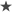# Shear correction factors for beams, plates and shells

Shear correction factors are very important for finite element models that assume that the transverse shear deformations are constant across the thickness of the elements. These factors are specially important when sandwich type materials are modeled and, in particular, when the shear modulus of the core are lower than the shear modulus of the face sheets.

Themes are: Bernoulli beam theory (excludes shear deformations), Timoshenko beam theory (includes shear deformations), shear stress distribution in rectangular beam cross sections (Jourawski's equation for parabolic shear stress distribution), shear strain energy, plate bending, Kirchhoff plate theory (excludes shear deformations) and Reissner-Mindlin plate theory (includes shear deformations).

# Constitutive relations for plane stress material with transverse shear deformations

Notes: here are some notes of constitutive relations, starting with linear elastic anisotropic material model. Notes focus on finding the constitutive relation for a plane stess material model that includes transverse shear deformation. This kind of material model is used in plates and shells that use an orthotropic material where stress sigma33 is assumed to be zero and includes shear modulus G23 and G13. Latex files of the .pdf document can be downloaded here.

# Basics of finite element analysis

FEM TP1: Weak formulations.

FEM TP2: Shape functions.

FEM TP3: Heat transfer.

FEM TP4: Linear elasticity.

FEM TP5: Unidimensional transient heat transfer analysis.

FEM TP6: Convection - Diffusion problem.

FEM TP7: Two dimensional heat transfer analysis - Heat exchanger.

# Finite element analysis - Solids

TP1: Non-linear system solution: convergence criteria, Newton-Rhapson, BFGS.

TP2: Total Lagrangian Formulation problem.

TP3: Von-mises elasto-plasticity problem.

# Basic notes on continuum mechanics

Continua: deformation gradient tensor, second piola-kirchhoff stress tensor, green-lagrange strain tensor.

Elasto-plasticity: Von Mises plasticity, hardening law, strain hardening modulus, perfect plasticity, kinematic hardening, radial return algorithm.

Plane strain and plain stress: the two material models.

Tensors: some theory about tensor algebra.